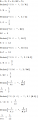# Step response of resistor-capacitor circuit

#### halfeclipse

Joined Feb 2, 2017
11
So the material in this assignment hasn't actually been covered in lecture yet and I'm going into this a little blind. So I'd be very appreciative if someone could sanity check my answer for the first part here as the remaining questions depend on getting this correct.I've got a capacitor circuit with a switch in it that goes from the state in the first image to the state in the second when the switch is flipped, and it would like me to derive the equations for the voltage across and current through the capacitor after the switch is flipped. in the interest of making my life easier, i convert that to the norton equivalentwhere Rth=R+Rt and It=Vt/Rth

Summing the currents at the top node gets me $$It=C(\frac{dv}{dt})+\frac{v}{Rth}$$ and I can solve that to get

$$v(t)=ItRth+(v(0)-ItRth)e^{(\frac{-t}{RthC}) for t>=0 similarly I can get the current \(i(t)=(It-\frac{v(0)}{R})e^{(\frac{-t}{RthC})$$

for t>0

The capacitor isn't connected to a source in the initial state so v(0)=0 and i(0)=0. Subbing that back in along with It=Vt/Rth gets me

$$v(t)=Vt-Vte^{(\frac{-t}{RthC}) t>=0 i(t)=(Vt/Rth)e^{(\frac{-t}{RthC}) t>0 i(t)=0 t<=0.$$

Have I done something horribly wrong here with my derivation or am I on the right track?\)

Last edited:

#### halfeclipse

Joined Feb 2, 2017
11
oh goody, timer ran out while latexing it up.

final equations should look like:

$$v(t)=Vt-Vte^{(\frac{-t}{RthC})$$ for t>=0

$$i(t)=(Vt/Rth)e^{(\frac{-t}{RthC})$$ for t>0

$$i(t)=0$$ for t=0

#### WBahn

Joined Mar 31, 2012
29,180
You have made a very common notational mistake.

1/RC is NOT the same as 1/(RC). This is because multiplication and division are of the same level of precedence and they are left associative, so

1/RC = ((1/R)·C) = C/R

You want to get in the habit of catching and correcting things like this because they WILL get you in trouble as so as you start trying to write programs or use spreadsheets. If you are used to writing 1/RC and interpreting it as 1/(R*C), then you will invariably write an equation in code as 1/R*C and then spend hours trying to figure out why it isn't producing valid results.

Other than that, ask yourself some sanity check questions.

First and foremost -- do the units work out? This includes the units in the argument to the exponential, which must be dimensionless since it is a transcendental function.

If you don't know already, then you expect the solution to an RC circuit to be a 1st order response, which is an exponential, so your solutions are at least of the correct form.

What do you know that v(t=0) and i(t=0) should be? Do your equations yield the expected values?

What do you know that v(t=oo) and i(t=oo) should be? Do your equations yield the expected values?

If your solutions are of the right form and pass these tests, then it is almost guaranteed that they are correct (not completely guaranteed because the time constant could be wrong).

#### WBahn

Joined Mar 31, 2012
29,180
oh goody, timer ran out while latexing it up.

final equations should look like:
You might latex it up some more using subscripts and scalable parens:

$$v(t)=V_t-V_te^{\(\frac{-t}{R_{th}C}$$\) for t>=0

$$i(t)=\(\frac{V_t}{R_{th}}$$e^{$$\frac{-t}{R_{th}C}$$\) for t>0

$$i(t)=0$$ for t=0

#### The Electrician

Joined Oct 9, 2007
2,936
You want to get in the habit of catching and correcting things like this because they WILL get you in trouble as so as you start trying to write programs or use spreadsheets. If you are used to writing 1/RC and interpreting it as 1/(R*C), then you will invariably write an equation in code as 1/R*C and then spend hours trying to figure out why it isn't producing valid results.
Something else for potential users of modern mathematical software to watch out for is this: simple concatenation of variables is often taken to mean multiplication; that is, R*C can also be written as a simple concatenation of R and C, but a space between R and C must be provided because RC is a simple variable on its own right, whereas R C is the same as R*C.

Some examples from Mathematica:#### MrAl

Joined Jun 17, 2014
10,621
Hi,

Yes good point. I use RC as a single variable in many cases because it makes life easier sometimes, but i always try to remember to make a note of this. So when i type for example:
T=1/RC

i also make the note:
RC=R*C

so it becomes clear that i really mean:
T=1/(R*C)

I also sometimes write small programs to calculate something about a circuit that has to be able to calculate a lot of points really fast so i end up taking out variable names such as:

atom R,C,T,RC (just some float variables using the keyword 'atom' for this particular language)

R=10000
C=0.01e-6

and then:
RC=R*C

and finally:
T=1/RC

It just makes so much sense to do this in a program so that it does not have to keep calculating R*C over and over again when the two are inseparable throughout the entire program anyway, as the usual calculations after that are always something like:
Vc=Vs*exp(-t/RC)

which i would have to enter into the program as:
Vc=Vs*exp(-t/(R*C))

which means an extra multiplication EVERY time it calls that function, or make the program less readable with:
Vc=Vs*exp(-t/R/C)

which not only means an extra division but also means it is a lot less readable from a programming and electrical standpoint.